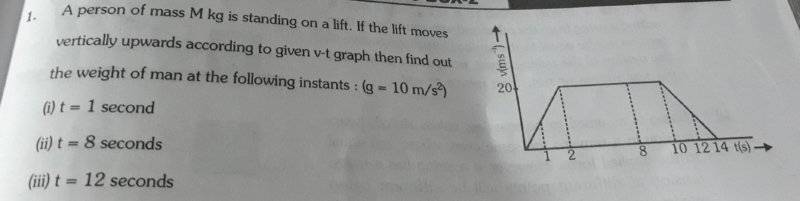# Instantaneous acceleration from Velocity-time graph

## Homework Statementhttps://imgur.com/a/bvcxjNy

## Homework Equations

Since the elevator is accelerating upwards,
N - mg = ma
or N = m(g+a) ------------ (1)

## The Attempt at a Solution

In order to get the accelerations at that instant, I drew a tangent at that point and used v2 - v1/t2 - t1 = a
and got;
for (i) t = 1s
v2 - v1/t2 - t1 = a;
20-10/2-1 = a
10 m/s^2 = a
Applying this to equation (1)
N = m(10+10) = 20m

(ii) Following the same steps as in (i)
20-20/8-2 = 0 m/s^2
N = m(10+0) = 10m

(iii) 10-20/12-10 = -10/5
a = -5m/s^2
N = m(10-5) = 5m

The answer matches the solution but I have a doubt about the process of arriving at instantaneous acceleration.
The tangent gives the instantaneous acceleration and we can use any points on the tangent to calculate to the acceleration.So for eg,

In (ii) above I used; v2 = 20, v1 = 20, t2 = 8 and t1 = 2 secs
But if instead of those I took v2 = 20, v1 = 0 t2 = 8 and t1 = 0 why does my answer comes out wrong?

## Answers and Replies

kuruman
Science Advisor
Homework Helper
Gold Member
Please place parentheses where they belong. The expression (v2-v1)/(t2-t1) gives the average acceleration over the time interval t1 to t2. When the slope is constant (straight line) the average acceleration is equal to the instantaneous acceleration. The slope is constant from 0 to 2 s, from 2 to 10 s and from 10 to 14 s. It is not constant from 0 to 8 s. Over that time interval the acceleration is changing so the expression gives the average and not the instantaneous value.

BvU
Science Advisor
Homework Helper
Please type the problem statement and post a picture, not a link (that's gone soon)

RPinPA
Science Advisor
Homework Helper
The tangent gives the instantaneous acceleration... But if instead of those I took v2 = 20, v1 = 0 t2 = 8 and t1 = 0 why does my answer comes out wrong?

Because those aren't two points on the same tangent. The line between those points cuts across the graph, it isn't tangent to it.

Since this graph consists of straight lines, the tangent in each segment is just a line coinciding with that straight line.

Please place parentheses where they belong. The expression (v2-v1)/(t2-t1) gives the average acceleration over the time interval t1 to t2. When the slope is constant (straight line) the average acceleration is equal to the instantaneous acceleration. The slope is constant from 0 to 2 s, from 2 to 10 s and from 10 to 14 s. It is not constant from 0 to 8 s. Over that time interval the acceleration is changing so the expression gives the average and not the instantaneous value.
Thank you so much!

SammyS
Staff Emeritus
Science Advisor
Homework Helper
Gold Member
Thank you so much!
As @BvU said, you should post any picture. Here's your's:Also:
You should type the complete problem statement (even if it's given in the posted image or in the thread title).

Your's should read:
A person of mass M (in kg) is standing on a lift. If the lift moves vertically upward, according to given v-t graph, then find out the weight of the man at the following instants: (g = 10m/s2).

(i) t = 1 seconds

(ii) t = 8 seconds

(iii) t = 12 seconds​